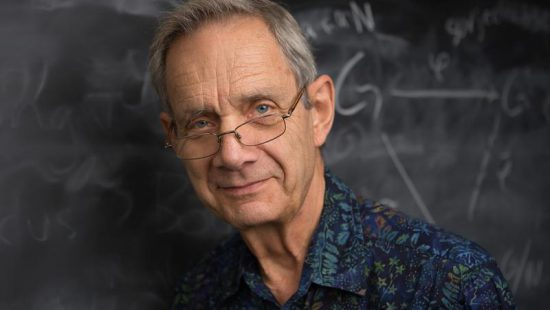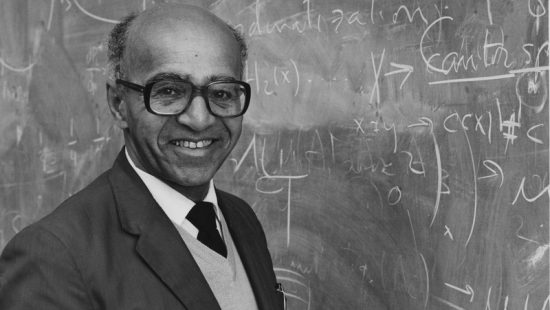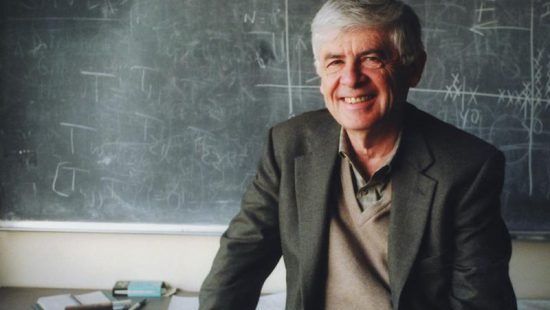# Alberto P. Calderon

• National Medal of Science
• Mathematics And Computer Science

For his ground-breaking work on singular integral operators leading to their application to important problems in partial differential equations, including his proof of uniqueness in the Cauchy problem, the Atiyah-Singer index theorem and the propagation of singularities of non-linear equations.

Alberto P. Calderón is regarded as one of this century’s leading mathematicians. His contributions have changed the way researchers approach a wide variety of areas in both pure mathematics and its applications to science.

His influence is felt strongly in a wide array of subjects from the abstract– harmonic analysis and partial differential equations—to more tangible fields, such as geophysics and tomography. Calderón is best known for his contributions in mathematical analysis, a large branch of mathematics that includes calculus, infinite series and the analysis of functions.

Alongside his mentor Antoni Zygmund, Calderón formulated a theory of singular integrals– mathematical objects that look infinite, but when interpreted properly are finite and well-behaved. Now known as the Calderón-Zygmund theory, Calderón showed how these singular integrals could be used to obtain estimates of solutions to equations in geometry and to analyze functions of complex variables. He also showed how singular integrals could provide entirely new ways to study partial differential equations, which continue to be widely used to solve problems in physics and engineering.

By Jenn Santisi

## Related Laureates### Michael Artin### David Blackwell